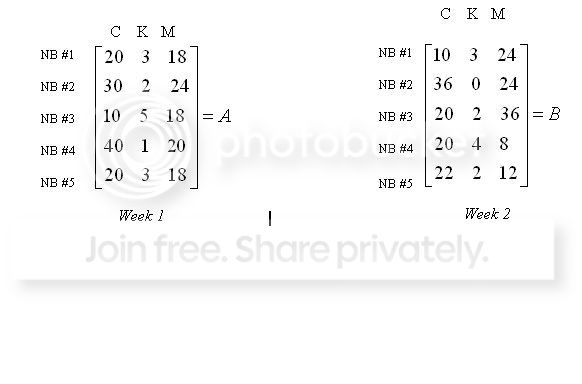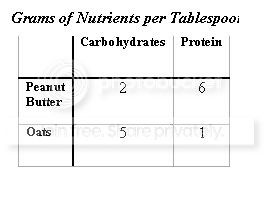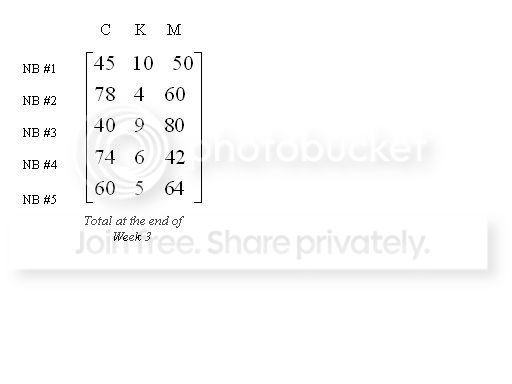• Location:
• Mood:
• Music:

# Need math help - word problems

I'm looking for someone who is good with math.  I am not good with matrices or word problems and these four problems on my homework are kiling me.  If someone could show me how to do any of these, it would be appreciated.

Five neighborhoods (NB) all want to raise money for a playground for their kids. The neighborhood that raises the most money will be able to choose the name of the park. To raise money, they all decide to have a bake sale and sell cookies (C), cakes (K), and muffins (M). They plan to sell their bake goods on Saturday morning for 3 weeks. Following are the results of the first 2 weeks. The numbers represent the number of cookies, cakes, and muffins sold.A. How much of each item had the neighborhoods sold by the end of the second week? Use matrices to solve the problem. Final answer must be given in matrix form. Show all   work to receive full credit.

B. Which team sold the most items at the end of the second week?

D. How much money did the neighborhoods make for their playground?

E. Which neighborhood gets to name the park?

2. Use augmented matrices to solve the following 2x2 systems of equations.  Show all work for full credit.  Final answer must be given in matrix form.
-5x+7=9
x+10y=21

x+5y=11
2x+10y=25

A group of students decides to sell pizzas to help raise money for their senior class trip. They sold pepperoni for \$12, sausage for \$10, and cheese for \$8. At the end of their sales the class sold a total of 600 pizzas and made \$5900. The students sold 175 more cheese pizzas than sausage pizzas. Set up a system of three equations and three unknowns, use an augmented matrix to solve, and show all work to receive full credit.

A. What are the three unknowns?

B. Write a separate equation representing each of the first three sentences.

C. Determine the augmented matrix that represents the three equations.

D. Solve for the matrix. Show all work to receive full credit.

E. How many of each type of pizza were sold?

A company’s employees are working to create a new energy bar. They would like the two key ingredients to be peanut butter and oats, and they want to make sure they have enough carbohydrates and protein in the bar to supply the athlete. They want a total of 31 carbohydrates and 23 grams of protein to make the bar sufficient. Using the following table, create a system of two equations and two unknowns to find how many tablespoons of each ingredient the bar will need. Solve the system of equations using matrices. Show all work to receive full credit.A. Write an equation for the total amount of carbohydrates.

B. Write an equation for the total amount of protein.

C. Determine the augmented matrix that represents the equations from A and B.

D. Solve for the matrix above. Show all work to receive full credit.

E. How many tablespoons of each will there need to be for the new energy bar?

C. By the end of the third week, the totals were as follows:If the neighborhoods makes a \$0.50 profit on each cookie, a \$5.00 profit on each cake, and a \$1.00 profit for each muffin they sell, how much profit did each neighborhood make? Final answer must be given in matrix form. Show all work to receive full credit.

Tags:
• Post a new comment

#### Error

Anonymous comments are disabled in this journal

default userpic